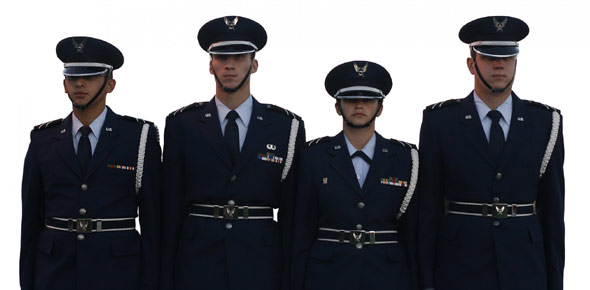# 3D151 Vol 2

100 Questions | Total Attempts: 539SettingsRelated Topics
• 1.
The process of condensation occurs when air particles are:
• A.

Pressurized, causing them to move closer together

• B.

Heated rapidly causing them to spread farther apart

• C.

Heated rapidly causing them to move closer together

• D.

Pressurized, causing them to be released to the atmosphere

• 2.
In hertz (Hz), what range of sound can the human ear detect?
• A.

200 to 3,000

• B.

20 to 20,000

• C.

20 to 200,000

• D.

Up to 2,600

• 3.
The loudness of a sound is closely related to what part of the sine wave?
• A.

Amplitude

• B.

Frequency

• C.

Tone

• D.

Pitch

• 4.
Noise may be introduced into speech signals by thermal agitation and:
• A.

Power variations

• B.

Amplitude variations

• C.

• D.

Molecular breakdown

• 5.
In hertz (Hz), what is the actual voice frequency band?
• A.

300 to 1,600

• B.

300 to 2,600

• C.

300 to 3,400

• D.

300 to 3,800

• 6.
Which models of end offices in the Digital Multiplex System (DMS) family are commonly used at Air Force bases to provide base telephone services?
• A.

DMS-100 and Meridian System Logic (MSL)-100

• B.

DMS-100/200 and MSL-100

• C.

DMS-100/200 and DMS-200

• D.

DMS-200 and MSL-100

• 7.
Which model of Digital Multiplex System (DMS)-100 family switches is installed in compact modular cabinets?
• A.

• B.

DMS-100

• C.

DMS-200

• D.

Meridian System Logic (MSL)-100

• 8.
What is the maximum number of ports that a Meridian System Logic (MSL)-100 will support?
• A.

10,000

• B.

30,000

• C.

100,000

• D.

300,000

• 9.
Which type link is normally used to interconnect two peripheral modules (PM) in the Digital Multiplex System (DMS)?
• A.

Digital signal (DS)-1

• B.

DS-30A

• C.

DS-32

• D.

T1A

• 10.
What major area is connected to the central side (C-side) of a network module (NM) in the Digital Multiplex System (DMS)?
• A.

Control node (CN)

• B.

Peripheral module (PM)

• C.

Message controller (MC)

• D.

Central control

• 11.
What is the mounting bracket called on which transmission or swtiching equipment is mounted in a Digital Multiplex System (DMS)?
• A.

Bay

• B.

Card

• C.

Shelf

• D.

Frame

• 12.
What contains bays of the same functional equipment?
• A.

Bay

• B.

Card

• C.

Shelf

• D.

Frame

• 13.
Which Digital Multiplex System (DMS) component uses 16-bit Motorola 68000 or 8-bit Intel microprocessors?
• A.

A device controller

• B.

The network message controller

• C.

A peripheral module's (PM) peripheral processor (PP)

• D.

The computing module's (CM) central processing unit (CPU)

• 14.
How many channels of data does each digital signal (DS) 30 speech link carry?
• A.

30

• B.

32

• C.

34

• D.

36

• 15.
Up to how many duplicated digital signals (DS)-30 links are used to connect a line group controller (LGC) to the network module (NM)?
• A.

6

• B.

8

• C.

16

• D.

24

• 16.
What type of digital signal (DS) links are used to connect the Digital Multiplex System (DMS)-Core and the DMS-Bus?
• A.

1

• B.

30

• C.

30A

• D.

512

• 17.
How many inter-message switch links (IMSL) can be configured in a SuperNode?
• A.

2

• B.

4

• C.

8

• D.

16

• 18.
Where are the system clocks located within the SuperNode?
• A.

Network module (NM)

• B.

Peripheral module (PM)

• C.

Digital Multiplex System (DMS)-Bus

• D.

DMS-Core

• 19.
What type of digital signal (DS) protocol is used as a message carrier between the Digital Multiplex System (DMS)-Bus and the network modules (NM) and input/output controller (IOC)?
• A.

1

• B.

3

• C.

30

• D.

512

• 20.
Where are the DMS-Core and DMS-Bus housed?
• A.

Line trunk controller (LTC)

• B.

Dual plane combined core (DPCC)

• C.

Multipoint controller (MC)

• D.

Multipoint control unit (MCU)

• 21.
• A.

Discrete amplitudes are assigned to the sampling pulses

• B.

A binary code number is assigned to the sample

• C.

The quantizer limits the amplitude of the pulses

• D.

The analog signal is band-limited

• 22.
In the pulse code modulation (PCM) process, changing the quantized samples into a binary word is called:
• A.

Encoding

• B.

Sampling

• C.

Quantization

• D.

Band limiting

• 23.
What is a method of putting many users on a single cable pair?
• A.

Time code multiplexing

• B.

Pulse code modulation

• C.

Pulse amplitude modulation

• D.

Time division multiplexing

• 24.
In the time division multiplexing (TDM) process, the rate of sampling must be equal to:
• A.

The lowest frequency sampled multiplied by the sampling rate per cycle

• B.

The highest frequency sampled multiplied by the sampling rate per cycle

• C.

Twice the lowest frequency sampled multiplied by the sampling rate per cycle

• D.

Twice the highest frequency sampled multiplied by the sampling rate per cycle

• 25.
Each 125 microsecond time period in the digital signal (DS) 30 signaling format (used within the voice network system) is called a:
• A.

Span

• B.

Frame

• C.

Channel

• D.

Snapshot